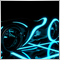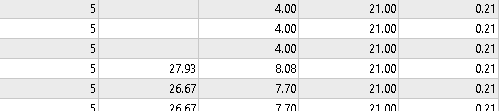# Backtest not showing profit factor1488

Hi There,

right now I am backtesting and optimizing an EA, that I work on for some time...

The problem now is: The Profit Factor of some optimized trades is not being shown.

Does someone know why?!

[second column is pf]I have a quite high pf at some trades, 27 aso.

Might there be a maximum pf the strategy tester is able to show, and not more?!

What exactly is the formula for getting to know the pf?! I think the profit and dd is important; how do I calculate the number of trades in?!

Thanks10084

That means profit factor is infinite.

Profit factor is calculated by dividing profits by losses.

So if losses are 0 then PF is infinite and is not shown1488

Tomas Rodriguez Doherty:

That means profit factor is infinite.

Profit factor is calculated by dividing profits by losses.

So if losses are 0 then PF is infinite and is not shown

Wow, thanks for the explanation.
I didn't saw that.

So, number of Trades does not change anything?!10084

Andreas Bauer:
Wow, thanks for the explanation.
I didn't saw that.

So, number of Trades does not change anything?!

No, the number of trades has nothing to do.if all trades are profitable then profit factor is always infinite independently from the number of trades. Cheers
To add comments, please log in or register